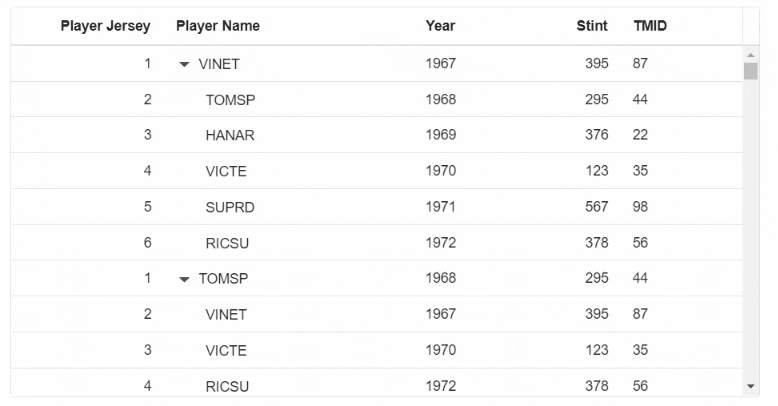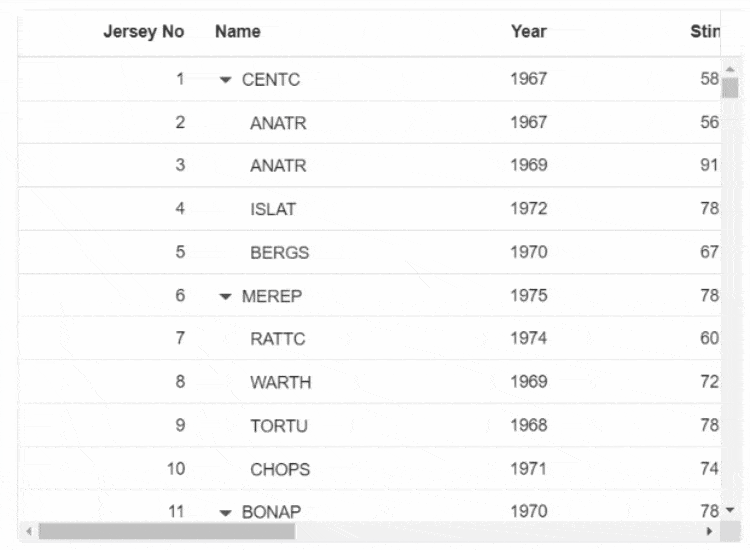# Virtualization in Blazor TreeGrid Component

24 Aug 202124 minutes to read

Tree Grid allows you to load large amount of data without performance degradation.

## Row Virtualization

Row virtualization allows you to load and render rows only in the content viewport. It is an alternative way of paging in which the rows will be appended while scrolling vertically. To setup the row virtualization, you need to define EnableVirtualization as true and content height by Height property.

The number of records displayed in the Tree Grid is determined implicitly by height of the content area and a buffer records will be maintained in the Tree Grid content in addition to the original set of rows.

Expand and Collapse state of any child record will be persisted.

``````@using TreeGridComponent.Data;
@using Syncfusion.Blazor.TreeGrid;
@using Syncfusion.Blazor.Grids;

<SfTreeGrid TValue="VirtualData" DataSource="@TreeGridData" ChildMapping="Children" EnableVirtualization="true" Height="350" TreeColumnIndex="1">
<TreeGridColumns>
</TreeGridColumns>
</SfTreeGrid>

@code{
public VirtualData[] TreeGridData { get; set; }

protected override void OnInitialized()
{
this.TreeGridData = VirtualData.GetVirtualData().ToArray();
}
}``````
``````namespace TreeGridComponent.Data {

public class VirtualData
{
public int? TaskID { get; set; }
public string FIELD1 { get; set; }
public int? FIELD2 { get; set; }
public int? FIELD3 { get; set; }
public int? FIELD4 { get; set; }
public List<VirtualData> Children { get; set; }

public static List<VirtualData> GetVirtualData()
{
List<VirtualData> DataCollection = new List<VirtualData>();

for (var i = 1; i <= 1000; i++)
{
VirtualData Parent1 = new VirtualData()
{
FIELD1 = "VINET",
FIELD2 = 1967,
FIELD3 = 395,
FIELD4 = 87,
Children = new List<VirtualData>()
};
VirtualData Child1 = new VirtualData()
{
FIELD1 = "TOMSP",
FIELD2 = 1968,
FIELD3 = 295,
FIELD4 = 44
};
VirtualData Child2 = new VirtualData()
{
FIELD1 = "HANAR",
FIELD2 = 1969,
FIELD3 = 376,
FIELD4 = 22
};
VirtualData Child3 = new VirtualData()
{
FIELD1 = "VICTE",
FIELD2 = 1970,
FIELD3 = 123,
FIELD4 = 35
};
VirtualData Child4 = new VirtualData()
{
FIELD1 = "SUPRD",
FIELD2 = 1971,
FIELD3 = 567,
FIELD4 = 98
};
VirtualData Child5 = new VirtualData()
{
FIELD1 = "RICSU",
FIELD2 = 1972,
FIELD3 = 378,
FIELD4 = 56
};
VirtualData Parent2 = new VirtualData()
{
FIELD1 = "TOMSP",
FIELD2 = 1968,
FIELD3 = 295,
FIELD4 = 44,
Children = new List<VirtualData>()
};
VirtualData Child6 = new VirtualData()
{
FIELD1 = "VINET",
FIELD2 = 1967,
FIELD3 = 395,
FIELD4 = 87
};
VirtualData Child7 = new VirtualData()
{
FIELD1 = "VICTE",
FIELD2 = 1970,
FIELD3 = 123,
FIELD4 = 35
};
VirtualData Child8 = new VirtualData()
{
FIELD1 = "RICSU",
FIELD2 = 1972,
FIELD3 = 378,
FIELD4 = 56
};
VirtualData Child9 = new VirtualData()
{
FIELD1 = "HANAR",
FIELD2 = 1969,
FIELD3 = 376,
FIELD4 = 22
};
VirtualData Child10 = new VirtualData()
{
FIELD1 = "SUPRD",
FIELD2 = 1971,
FIELD3 = 567,
FIELD4 = 98
};

}
return DataCollection;
}
}
}``````

The following output is displayed as a result of the above code example.## Column Virtualization

Column virtualization allows you to virtualize columns. It will render columns which are in the viewport. You can scroll horizontally to view more columns.

To setup the column virtualization, set the
EnableVirtualization and
EnableColumnVirtualization properties as true.

``````@using Syncfusion.Blazor.Grids
@using Syncfusion.Blazor.TreeGrid;

<SfTreeGrid DataSource="@TreeData" IdMapping="TaskID" TreeColumnIndex="1" ParentIdMapping="ParentID" Height="400" Width="600" EnableHover="false" EnableVirtualization="true" EnableColumnVirtualization="true">
<TreeGridPageSettings PageSize="40"></TreeGridPageSettings>
<TreeGridColumns>
</TreeGridColumns>
</SfTreeGrid>

@code{
public List<VirtualData> TreeData { get; set; }
protected override void OnInitialized()
{
this.TreeData = VirtualData.GetTreeVirtualData().ToList();
}

public class VirtualData
{
public int TaskID { get; set; }
public string FIELD1 { get; set; }
public int FIELD2 { get; set; }
public int FIELD3 { get; set; }
public int FIELD4 { get; set; }
public int FIELD5 { get; set; }
public int FIELD6 { get; set; }
public int Field7 { get; set; }
public int Field8 { get; set; }
public int Field9 { get; set; }
public int? ParentID { get; set; }
public static List<VirtualData> GetTreeVirtualData()
{
string[] Names = new string[] { "VINET", "TOMSP", "HANAR", "VICTE", "SUPRD", "HANAR", "CHOPS", "RICSU", "WELLI", "HILAA", "ERNSH", "CENTC",
"OTTIK", "QUEDE", "RATTC", "ERNSH", "FOLKO", "BLONP", "WARTH", "FRANK", "GROSR", "WHITC", "WARTH", "SPLIR", "RATTC", "QUICK", "VINET",
"MAGAA", "TORTU", "MORGK", "BERGS", "LEHMS", "BERGS", "ROMEY", "ROMEY", "LILAS", "LEHMS", "QUICK", "QUICK", "RICAR", "REGGC", "BSBEV",
"COMMI", "QUEDE", "TRADH", "TORTU", "RATTC", "VINET", "LILAS", "BLONP", "HUNGO", "RICAR", "MAGAA", "WANDK", "SUPRD", "GODOS", "TORTU",
"OLDWO", "ROMEY", "LONEP", "ANATR", "HUNGO", "THEBI", "DUMON", "WANDK", "QUICK", "RATTC", "ISLAT", "RATTC", "LONEP", "ISLAT", "TORTU",
"WARTH", "ISLAT", "PERIC", "KOENE", "SAVEA", "KOENE", "BOLID", "FOLKO", "FURIB", "SPLIR", "LILAS", "BONAP", "MEREP", "WARTH", "VICTE",
"HUNGO", "PRINI", "FRANK", "OLDWO", "MEREP", "BONAP", "SIMOB", "FRANK", "LEHMS", "WHITC", "QUICK", "RATTC", "FAMIA" };
List<VirtualData> DataCollection = new List<VirtualData>();
Random random = new Random();
var RecordID = 0;
for (var i = 1; i <= 2000; i++)
{
var name = random.Next(0, 100);
VirtualData Parent = new VirtualData()
{
FIELD1 = Names[name],
FIELD2 = 1967 + random.Next(0, 10),
FIELD3 = 395 + random.Next(100, 600),
FIELD4 = 87 + random.Next(50, 250),
FIELD5 = 410 + random.Next(100, 600),
FIELD6 = 67 + random.Next(50, 250),
Field7 = (int)Math.Floor(random.NextDouble() * 100),
Field8 = (int)Math.Floor(random.NextDouble() * 10),
Field9 = (int)Math.Floor(random.NextDouble() * 10),
ParentID = null
};
for (var j = 1; j <= 4; j++)
{
var childName = random.Next(0, 100);
{
FIELD1 = Names[childName],
FIELD2 = 1967 + random.Next(0, 10),
FIELD3 = 395 + random.Next(100, 600),
FIELD4 = 87 + random.Next(50, 250),
FIELD5 = 410 + random.Next(100, 600),
FIELD6 = 67 + random.Next(50, 250),
Field7 = (int)Math.Floor(random.NextDouble() * 100),
Field8 = (int)Math.Floor(random.NextDouble() * 10),
Field9 = (int)Math.Floor(random.NextDouble() * 10),
});
}
}
return DataCollection;

}
}
}``````

Column’s Width is required for column virtualization. If column’s width is not defined then Tree Grid will consider its value as 200px.

The following GIF represent a Tree Grid with Column virtualization.## Limitations for Virtualization

• While using column virtualization, column width should be in the pixel. Percentage values are not accepted.
• Due to the element height limitation in browsers, the maximum number of records loaded by the tree grid is limited by the browser capability.
• Cell selection will not be persisted in both row and column virtualization.
• Stacked Header is not compatible with detail template.
• Virtual scrolling is not compatible with detail template.
• Row count of the page does not depend on the PageSize property of the TreeGridPageSettings. Row count for the page is determined by the Height given to the Tree Grid.
• The virtual height of the tree grid content is calculated using the row height and total number of records in the data source and hence features which changes row height such as text wrapping are not supported. If you want to increase the row height to accommodate the content then you can specify the row height as below to ensure all the table rows are in same height.
• Programmatic selection using the SelectRows method is not supported in virtual scrolling.
• Frozen column feature is not supported with Virtual Scrolling.